# Precalculus : Fundamental Trigonometric Identities

## Example Questions

### Example Question #51 : Fundamental Trigonometric Identities

Evaluate the following.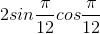Possible Answers: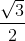Correct answer:Explanation:

Here we use the double angle identity for sine, which is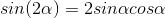We can rewrite the originial expression as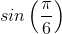using the double angle identity.

From here we can calculate that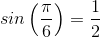.

### Example Question #52 : Fundamental Trigonometric Identities

Evaluate the following expression.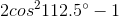Possible Answers: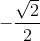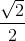Correct answer:Explanation:

One of the double angle formuals for cosine is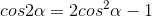We can use this double angle formula for cosine to rewrite the expression given as the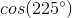because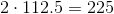and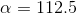.

We can then calculate that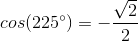.

### Example Question #53 : Fundamental Trigonometric Identities

Evaluate the following.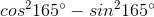Possible Answers:Correct answer:Explanation:

Here we can use another double angle formula for cosine,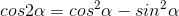.

Here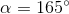, and so we can use the double angle formula for cosine to rewrite the expression as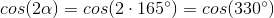.

From here we just recognize that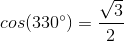.

### Example Question #54 : Fundamental Trigonometric Identities

Evaluate the following expression.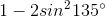Possible Answers: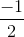Correct answer:Explanation:

Here we can use yet another double angle formula for cosine: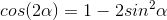.

First, realize that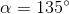.

Next, plug this in to the double angle formula to find that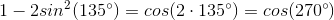.

Here we recognize that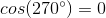### Example Question #55 : Fundamental Trigonometric Identities

Simplify the following. Leave your answer in terms of a trigonometric function.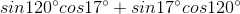Possible Answers: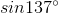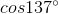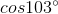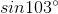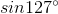Correct answer:Explanation:

This is a quick test of being able to recall the angle sum formula for sine.

Since,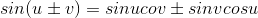, and here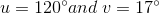, we can rewrite the expression as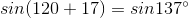.

### Example Question #56 : Fundamental Trigonometric Identities

Which of the following is equivalent to the expression: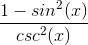Possible Answers: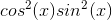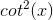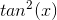Correct answer:Explanation:

Which of the following is equivalent to the following expression?Recall our Pythagorean trig identity: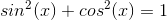It can be rearranged to look just like our numerator: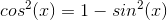So go ahead and change our original expression to: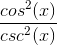Then recall the definition of cosecant: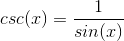So our original expression can be rewritten as: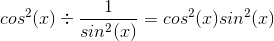So our answer is:### Example Question #57 : Fundamental Trigonometric Identities

Which of the following trigonometric identities is INCORRECT?

Possible Answers: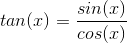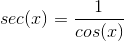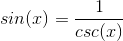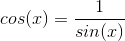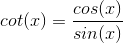Correct answer:Explanation:

Cosine and sine are not reciprocal functions.and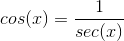### Example Question #58 : Fundamental Trigonometric Identities

Using the trigonometric identities prove whether the following is valid: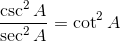Possible Answers:

False

Uncertain

True

Only in the range of: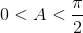Only in the range of: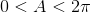Correct answer:

True

Explanation:

We begin with the left-hand side of the equation and utilize basic trigonometric identities, beginning with converting the inverse functions to their corresponding base functions: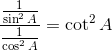Next, we rewrite the fractional division in order to simplify the equation: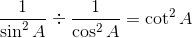In fractional division we multiply by the reciprocal as follows: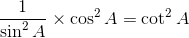If we reduce the fraction using basic identities we see that the equivalence is proven: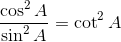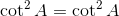### Example Question #59 : Fundamental Trigonometric Identities

Which of the following identities is incorrect?

Possible Answers: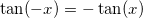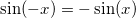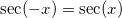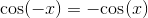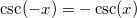Correct answer:Explanation:

The true identity is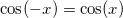because cosine is an even function.

### Example Question #60 : Fundamental Trigonometric Identities

State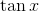in terms of sine and cosine.

Possible Answers: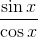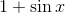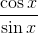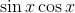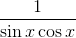Correct answer:Explanation:

The definition of tangent is sine divided by cosine.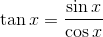### All Precalculus Resources